• PowerBI的查询编辑器使用Power Query M公式语言来定义查询模型，它是一种富有表现力的数据糅合（Mashup）语言，一个M查询可以计算（Evalute）一个表达式，得到一个值。 对于开发者来说，M公式常用于Power Query编辑...

PowerBI的查询编辑器使用Power Query M公式语言来定义查询模型，它是一种富有表现力的数据糅合（Mashup）语言，一个M查询可以计算（Evalute）一个表达式，得到一个值。
对于开发者来说，M公式常用于Power Query编辑器中，用于添加计算列，并对数据进行处理。开发者只需要知道简单的Power Query M公式函数，就可以利用PowerBI提供的UI界面来实现数据的处理。
一，访问数据
PowerBI极大地简化了M公式的使用难度，使得开发人员可以使用UI来修改数据模型。
访问数据得函数，例如，Sql.Database 函数，从SQL Server实例中执行TSQL查询脚本返回表值。

二，添加列
1，添加用户列
根据业务需要，开发工程师填写表达式，根据现有的数据列和公式，把结果存储到数据模型中。
添加的M查询，只能用于单个查询中，当M公式引用右侧的可用列时，需要使用中括号[]来指定，比如下面的 [Date]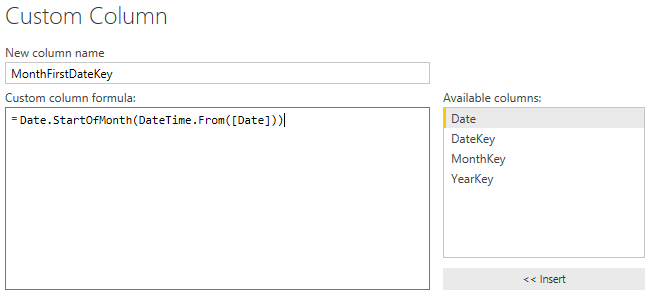2，添加条件列
在单个查询中，根据列的值的不同，使用不同的表达式，这是条件列的使用场景，Value字段，可以是参数（Parameter）、常量值、或者是数据列（Column）。PowerBI根据条件表达式计算新值，并添加到数据模型中。参考文档：
Power Query M function reference
Expressions, values, and let expression
Add a custom column in Power BI Desktop

转载于:https://www.cnblogs.com/ljhdo/p/4953714.html
展开全文• Latex之数学公式基本使用格式 一. 数学公式格式背景介绍 TEX有两种数学公式，一种是夹杂行文段落中的公式，一般称为行内(inline) 数学公式，或...两种公式使用不同的方式进入数学模式。TEX中，行内数学公式一...
Latex之数学公式基本使用格式

一. 数学公式格式背景介绍

TEX有两种数学公式，一种是夹杂在行文段落中的公式，一般称为行内(inline) 数学公式，或正文(in-tex:t)数学公式；另一种就是像单独占据整行居中展示出来的，称为显示(displayed)数学公式（或行间公式、列表公式），显示数学环境更适合表现更复杂的数学内容。两种公式使用不同的方式进入数学模式。在TEX中，行内数学公式一般在前后单给美元符号表示。

A. 行内(inline)数学公式

例如：交换律 (方法一) 是：a + b = b + a, 如 1 + 2 = 2 + 1 。说明：因此，在排版数学公式时，即使是没有任何特殊符号的算式 I+I, 或者简单地一个字母变总 x, 也要进入数学模式，使用 1 + 1 、x, 而不应该用排版普通文字的方式搞成 1+1 、X。除了使用单个美元符号，在 latex中还额外定义了命令格式与环境格式的方式输入行内公式，即使用命令＼（和＼）或是 math 环境括起一个行内数学公式，如 a+b 也可以写成 a+b或是 a + b。这两种形式提供了更好的错误检查，并且可以更明确地看出公式的开始与结束，也不容易混淆。但因为输人起来比较复杂，多数人更偏爱直接使用传统的＄表示行内数学公式。

B. 显示数学公式

显示数学公式也有多种方式输人。基本的显示公式是不带编号的，在 TEX 中可以用连续两个美元符号＄＄．．．＄＄界定。同样，LATEX也定义了命令形式和环境形式的输入方法，即用＼ [（和＼］命令或是displaymath 环境括起一个显示数学公式，例如：a + b = b + c,如1 + 2 = 2 + 1 = 3.虽然并非必须，但最好在源代码中就把单独占据一行的显示公式放在单独的行内．使代码更清晰。推荐的方式是使用＼［．．．＼］。＄＄．．．＄＄会产生不良的间距，缺少错误检查，并且不能正确处理 fleqn 等文档选项 [2671,应该避免使用，而 displaymath 环境又可能显得冗长。值得注意的是，显示公式后面如果有标点符号，应该放在数学环境内部，紧接着公式。而且因为数学模式下不能使用汉字，所以一般就使用西文的半角标点。这是说明一下 msmath 宏包，它提供的 text 命令就可以用来在数学公式中插入文字，例如 被减数 − 减数 = 差.

总结

1.
LATEX 使用一种特有的模式来排版数学 (mathematics) 公式。数学公式允许以行间形式排版在一个段落之中，也可以以独立形式排版，此时段落可能会被拆开。处于段内的数学文本要放在反斜杠之间，＄和＄之间，或者 begin(math) 与 end(math) 之间。
2.
当你希望把自己的一些较长的数学方程或是公式单独的放在段落之外的时候，那么你最好显示 (display) 它们，而不要拆开此段落。为此，你可以把它们放在反斜杠之间，或者 begin(displaymath) 与 end(displaymath)
之间。
3.
如果你希望 LATEX 给你的方程编上号，你可以使用 equation 环境。然后你就可以用 label 来给一个方程加上标签并在文中的某处用 ref 或amsmath 宏包中的 eqref 命令来引用它。

二. 一些常用的数学公式范例

01.平方

1：代码

 % 平方
\begin{flushleft}
1. 平方\\
\end{flushleft}
$c^{2} = a^{2} + b^{2}$

2： 结果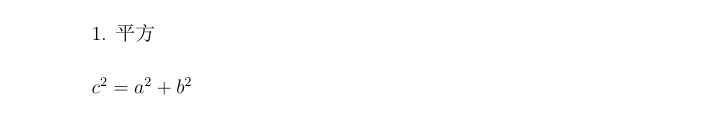02.和

1：代码

 % 和
\begin{flushleft}
2. 和\\
\end{flushleft}
$a +b =c$

2：结果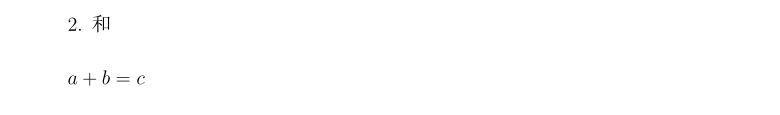03.极限

1：代码

% 极限1
\begin{flushleft}
2. 极限1\\
\end{flushleft}
$lim_{n \__\infty} \sum_{k=1}^n \frac{1}{k^2} = \frac{\pi^2}{6}$

 % 极限2
\begin{flushleft}
3. 极限2\\
\end{flushleft}

\begin{flushleft}
\begin{displaymath}
\lim_{n \to \infty}
\sum_{k=1}^n \frac{1}{k^2}
= \frac{\pi^2}{6}
\end{displaymath}
\end{flushleft}

2：结果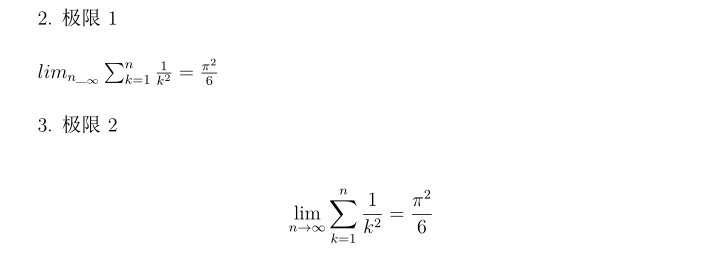04.符号表示

1：代码

% 符号表示
\begin{flushleft}
4. 符号表示\\
\end{flushleft}
\begin{displaymath}
\forall x \in \mathbf{R}:
\end{displaymath}

\begin{displaymath}
\textrm{for all } x \in\mathbf{R}
\end{displaymath}

% 不等式
\begin{flushleft}

2：结果05.不等式

1：代码

% 不等式
\begin{flushleft}
5. 不等式\\
\end{flushleft}
\begin{equation}
a^x +y \neq a^{x+y}
\end{equation}

2：结果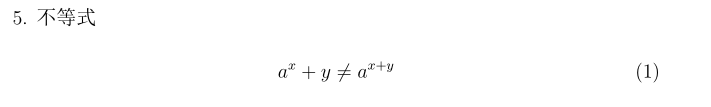06.数学公式的基本元素

1：代码

% 数学公式的基本元素
\begin{flushleft}
6. 数学公式的基本元素\\
\end{flushleft}

$\lambda, \xi , \pi, \mu, \Phi, \Omega$\\
指数和下标可以能过使用 $\^$ 和$\_$ 两个符号来指定。

$a_{1}$ \qquad $x^{2}$ \qquad
$e^{-\alpha t}$ \qquad $a^{3}_{i j}$\\
$e^{x^2} \neq {e^x}^2$

2：结果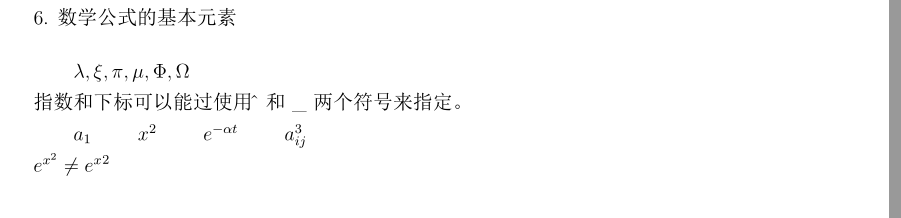07.平方根/font>

1：代码

% 平方根
\begin{flushleft}
6. 平方根\\
\end{flushleft}
$\sqrt{x}$ \qquad
$\sqrt{x^{2}+\sqrt{y}}$ \qquad
$\sqrt{2}$\$3pt]\\ \surd[x^2 +y^2] 2：结果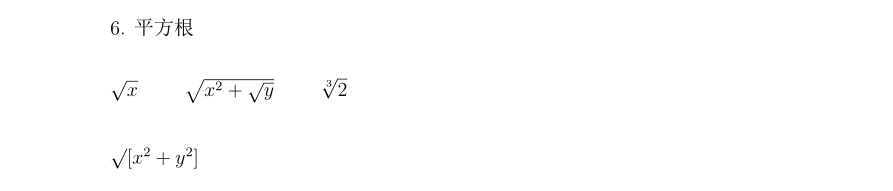08.水平线 1：代码 % 水平线 \begin{flushleft} 7. 水平线\\ \end{flushleft} \overline{m+n}\\ \underbrace{a+b+\cdots +z}_{26} 2：结果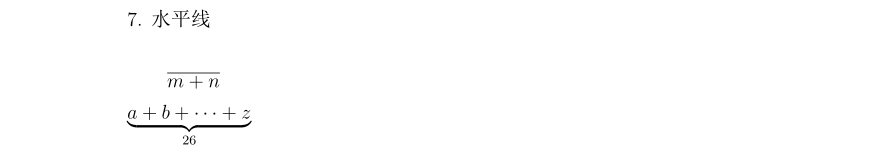09.函数 1：代码 % 函数 \begin{flushleft} 8. 函数\\ \end{flushleft} \begin{displaymath} y=x^{2}\qquad y'=2x\qquad y''=2 \end{displaymath} 2：结果10.向量 1：代码 % 向量 \begin{flushleft} 9. 向量\\ \end{flushleft} \begin{displaymath} \vec a\quad\overrightarrow{AB} \end{displaymath} 2：结果11.点号（用来分清公式） 1：代码 % 点号（用来分清公式） \begin{flushleft} 10. 点号（用来分清公式）\\ \end{flushleft} \begin{displaymath} v = {\sigma}_1 \cdot {\sigma}_2{\tau}_1 \cdot {\tau}_2 \end{displaymath} 2：结果12.log 等类似的函数名 1：代码 % log等类似的函数名 \begin{flushleft} 11. log等类似的函数名\\ \end{flushleft} \[\lim_{x \rightarrow 0}\frac{\sin x}{x}=1$

log 等类似的函数名通常是用直立字体，而不是如同变量一样用斜体，因此LATEX 提供了以下的命令来排版这些最重要的函数名：
\begin{figure}[H]
\centering % 居中
\includegraphics[height=5cm,width=14cm]{log.png}% 设置图片的高和宽以及指定要导入的图片文件名
\caption{log等类似函数名}% 生成图片标题
\label{1}% 是给这个标题定义一个供latex本身识别的编号, 方便其它地方引用
\end{figure}

2：结果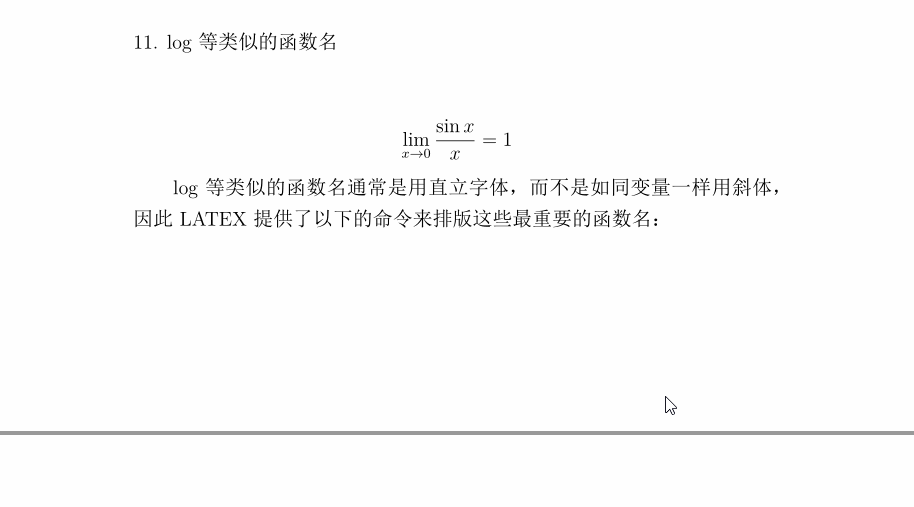13.上下分式可以用\frac{…}{…}命名得到

1：代码

%  上下分式可以用\frac{...}{...}命名得到
\begin{flushleft}
12. 上下分式\\
\end{flushleft}
$1\frac{1}{2}$ hours
\begin{displaymath}
x^{1/2}
\end{displaymath}

2：结果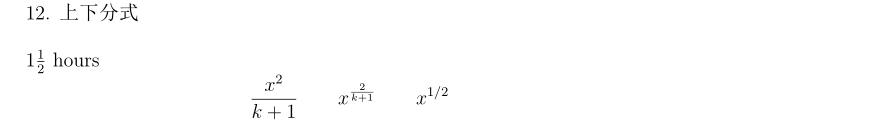14.二项系数（使用 amsmath 宏包中的 binom 命名

1：代码

%  二项系数（使用amsmath宏包中的\binom命名）
\begin{flushleft}
13. 二项系数（使用amsmath宏包中的binom命名）\\
\end{flushleft}
\begin{displaymath}
\end{displaymath}
对于二元关系，有时候你需要到把符号互相堆积起来。\ stackrel 命令会把其第一个参数中的符号以上标大小放在第二个上面，而第二个符号则以正常的位置摆放。
\begin{displaymath}
\int f_N(x) \stackrel{!}{=}1
\end{displaymath}

积分号(integral operator) 可以用\  int 产生，求和号(sum operator) 用\ sum命令，而乘积号(product operator) 要用\ prod 命令。上限和下限用$\^$ 和$\_$ 来指定，如同上标与下标一样。
\begin{displaymath}
\prod_\epsilon
\end{displaymath}

为了更好的控制一个复杂表达式中指标的放置，amsmath 提供了两个额外的工具：\ substack 命令和subarray 环境：
\begin{displaymath}
\sum_{\substack{0<i<n \\ 1<j<m}}
P(i,j) =
\sum_{\begin{subarray}{1}
i \in I\\1<j<m
\end{subarray} }    Q(i, j)
\end{displaymath}

2：结果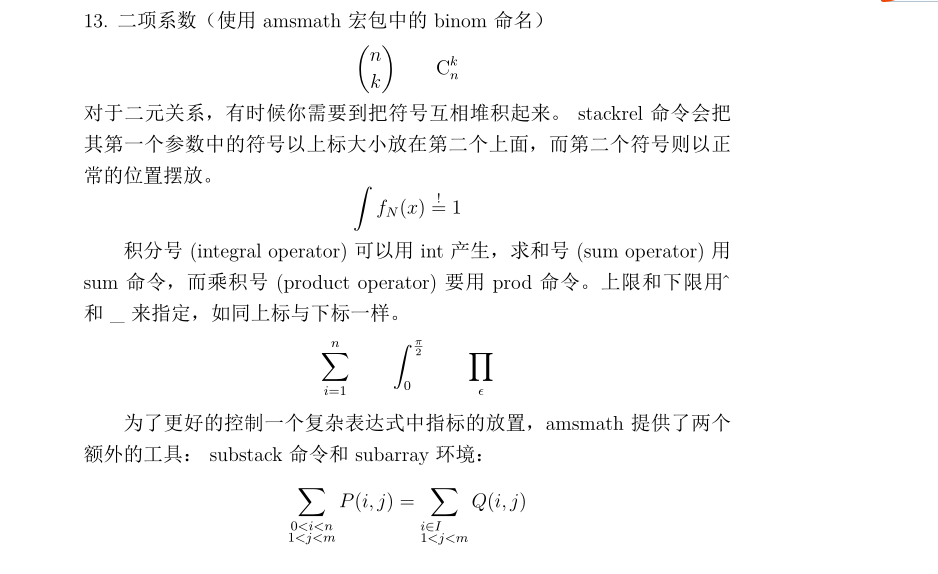15. 各种各样的括号

1：代码

% 各种各种的括号
\begin{flushleft}
14. 各种各样的括号\\
\end{flushleft}
\begin{displaymath}
{a,b,c}\neq\{a,b,c\}
\end{displaymath}

如果你在某个左定界符前放一个\ left 命令或是在某个右定界符前放一个\ right 命令，TEX 将会自动决定这对定界符的大小。请注意，你必须为每个\ left 命令配对相应的\ right 命令，而且只有在左右定界符被排版在同一行时才会获得正确的大小尺寸。如果你不想使用任何右定界符，使用看不见的‘\ right.’ 即可！
\begin{displaymath}
1 + \left(\frac{1}{1-x^{2}}
\right)^3
\end{displaymath}

有很多命令可以实现在公式中插入三点列(three dots)。\ ldots 得到在基线上的点列而\ cdots 是上下居中的点列。另外，还有\ vdots 命令产生竖直的点列，\ ddots 产生对角线的点列。
\begin{displaymath}
x_{1}+\cdots +x_{n}
\end{displaymath}

2：结果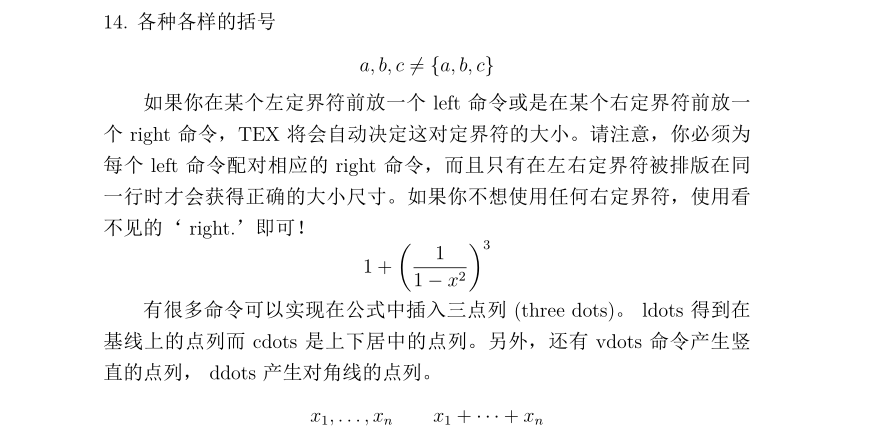16. 数学空格

1：代码

% 数学空格
\begin{flushleft}
15. 数学空格\\
\end{flushleft}

\newcommand{\ud}{\mathrm{d}}
\begin{displaymath}
\int\!\!\!\int_{D} g(x,y)
\, \ud x\, \ud y
\end{displaymath}
\begin{displaymath}
\int\int_{D} g(x,y)\ud x \ud y
\end{displaymath}

2：结果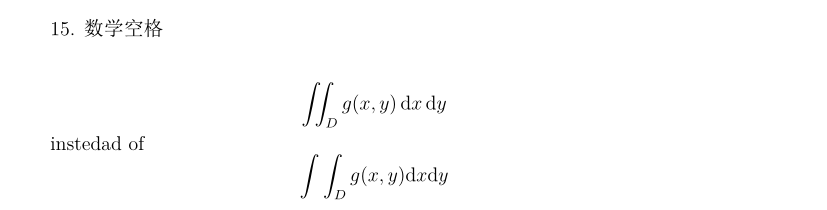17. 垂直取齐

1：代码

% 垂直取齐
\begin{flushleft}
16. 垂直取齐\\
\end{flushleft}
要排版数组，使用array 环境。它的使用与tabular 环境有些类似。双斜杠命令可用来断行。
\begin{displaymath}
\mathbf{X} =
\left(\begin{array}{ccc}
x_{11} & x_{12} & \ldots \\
x_{21} & x_{22} & \ldots \\
\vdots &\vdots & \ddots
\end{array} \right)
\end{displaymath}

array 环境也可以用来排版这样的表达式，表达式中使用一个“.” 作为其隐藏的\ right 定界符。
\begin{displaymath}
y = \left\{ \begin{array}{ll}
a & \textrm{if $d>c$}\\
b+x & \textrm{int the moring}\\
l & \textrm{all day long}
\end{array} \right.
\end{displaymath}

对于跨行的长公式或是方程组(equation system)，你可以使用eqnarray和eqnarray* 环境来替代equation 环境。在eqnarray 环境中每一行都有一个等式编号。eqnarray* 则不添加编号。eqnarray 和eqnarray* 环境的用法与一个{rcl} 形式的3 列表格相类似，这里中间一列可以用来放等号，不等号，或者是其他你选择的符号。双斜杠 命令可以断行。
\begin{eqnarray}
f(x) & = & \cos x \\
f’(x) & = & -\sin x \\
\int_{0}^{x} f(y)dy &
= & \sin x
\end{eqnarray}
注意，这里等号两边空白都有些大。“\ setlength \ arraycolsep(2pt)” 可以调小它，比如在下一个例子里。长等式不能被分成合适的小段。作者必须指定在哪里断且如何缩进。以下两方法是最常用的。
% 第一种
{\setlength\arraycolsep{2pt}
\begin{eqnarray}
\sin x & = & x -\frac{x^{3}}{3!}+\frac{x^{5}}{5!}-{}
\nonumber\\
&& {}-\frac{x^{7}}{7!}+{}\cdots
\end{eqnarray}}
% 第二种
\begin{eqnarray}
\lefteqn{ \cos x = 1-\frac{x^{2}}{2!} +{} }
\nonumber\\
& & {}+\frac{x^{4}}{4!}-\frac{x^{6}}{6!}+{}\cdots
\end{eqnarray}


2：结果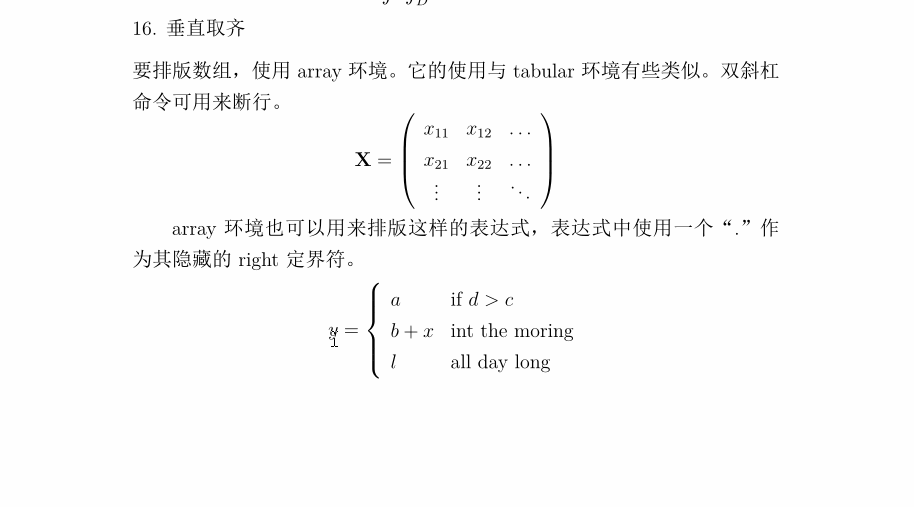18. 虚位

1：代码

 % 虚位
\begin{flushleft}
17. 虚位\\
\end{flushleft}
我们看不见虚位（phantom，也有幻影的意思），但是在许多人的头脑中它们依然占有一定的位置。LATEX 中也一样。我们可以使用它来实现一些有趣的小技巧。当使用$\^$ 和$\_$ 时，LATEX 对文本的垂直对齐有时显得太过于自作多情。使用\ phantom 命令你可以给不在最终输出中显示的字符保留位置。理解此意的最好方法是看下面的例子。
% 方法一
\begin{displaymath}
{}^{12}_{\phantom{1}6}\textrm{C}
{}^{12}_{6}\textrm{C}
\end{displaymath}

% 方法二
\begin{displaymath}
\Gamma_{ij}^{\phantom{ij}k}
\Gamma_{ij}^{k}
\end{displaymath}

% 数学字体尺寸
\begin{flushleft}

2：结果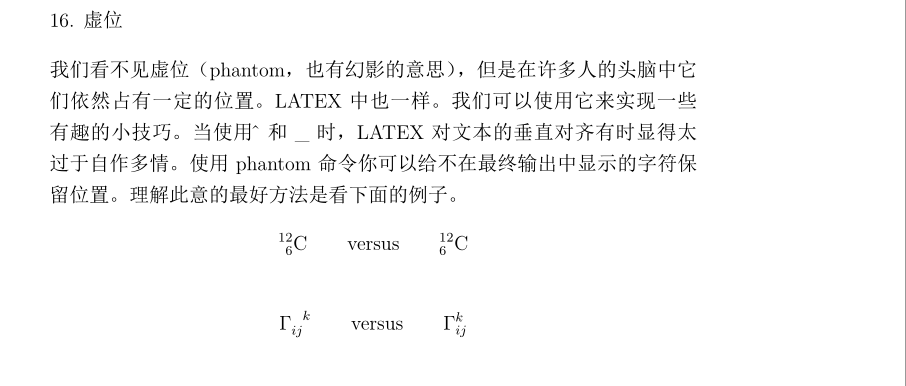19. 数学字体尺寸

1：代码

% 数学字体尺寸
\begin{flushleft}
18. 数学字体尺寸\\
\end{flushleft}
在数学模式中，TEX 根据上下文选择字体大小。例如，上标会排版成较小的字体。如果你想要把等式的一部分排版成罗马字体，不要用\ textrm 命令，只因\ textrm 会暂时切换到文本模式，而此时字体大小切换机制将不起作用。使用\ mathrm 来保持字体大小切换机制的正常。但是要小心，\ mathrm 只对较短的项有效。空格依然无效而且重音符号也不起作用。

\begin{equation}
2^{ \mathrm{nd}}
\end{equation}
有时你仍需告诉LATEX 正确的字体大小。在数学模式中，可用以下四个命令来设定：$\ displaystyle (123), \ textstyle (123), \ scriptstyle (123) and\ scriptscriptstyle (123)$. 改变样式也会影响到上下限的显示方式。
\begin{displaymath}
\frac{\displaystyle\sum_{i=1}^n(x_i-\overline x)(y_i-\overline y)}{\displaystyle\biggl[\sum_{i=1}^n(x_i-\overline x)^2\sum_{i=1}^n(y_i-\overline y)^2\biggr]^{1/2}}
\end{displaymath}

% 粗体符号
\begin{flushleft}

2：结果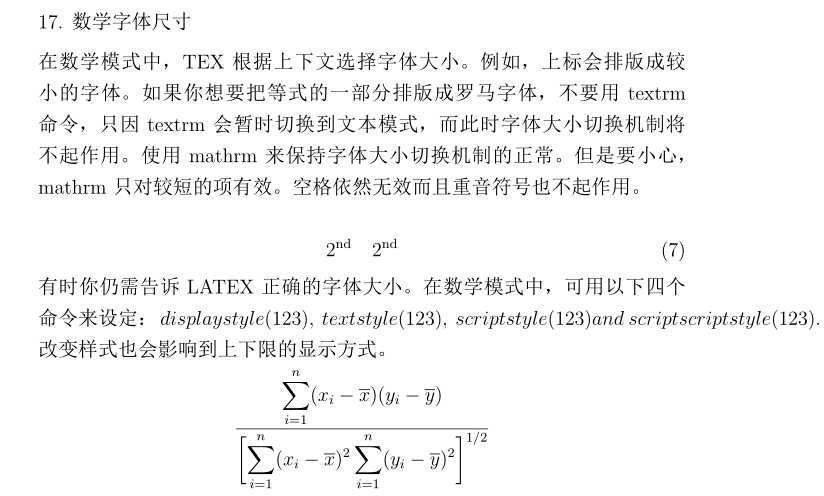20. 粗体符号

1：代码

% 粗体符号
\begin{flushleft}
19. 粗体符号\\
\end{flushleft}

在LATEX 中要得到粗体符号相当的不容易；这也许是故意设置的，以防业余水平的排版者过度的使用它们。字体变换命令\ mathbf 可得到粗体字母，但是得到的是罗马体（直立的）而数学符号通常要求是斜体。还有一个\ boldmath 命令，但是它只能用在数学模式之外。它不仅作用于字母也作用于符号。
\begin{displaymath}
\mbox{\boldmath $\mu, M$}
\end{displaymath}
请注意，逗号也成粗体了，这也许不是所需的。使用amsbsy 宏包（包含在amsmath 中）或tool 宏包集中的bm 将会便利许多，因为它们包含一个叫\ boldsymbol 的命令
\begin{displaymath}
\boldsymbol{\mu}, \boldsymbol{M}
\end{displaymath}

2：结果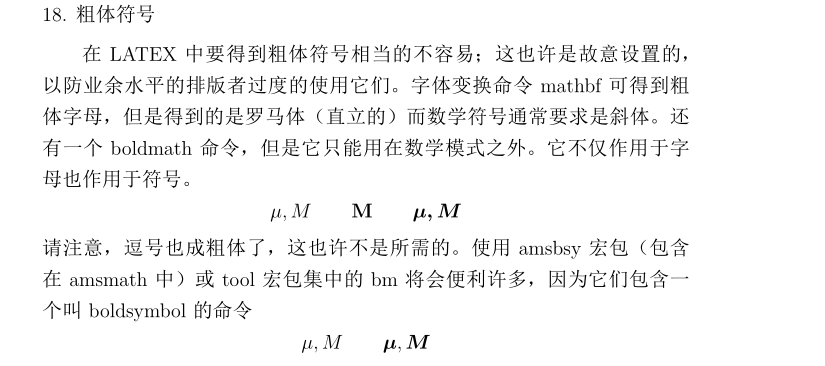21. 数学符号表

1：代码

% 数学符号表
\begin{flushleft}
18. 数学符号表\\
\end{flushleft}
要使用数学符号表，必须在导言区先载入amssymb宏包而行系统中安装了AMS数学字体。如果系统中没有安装AMS宏包和字体，查阅：
\url{https://www.ctan.org/tex-archive/macros/latex/required/amslatex}

2：结果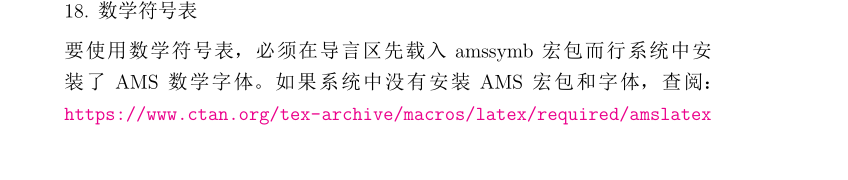三. 说明

对于此篇文档生成的pdf获取路径：数学公式基本使用格式(pdf)
对于此篇文档编写的.tex格式获取路径：数学公式基本使用(.tex格式内涵完整代码)
展开全文latex 基本操作
• 其次，点击“条件格式”，然后选择“新建规则”——“使用公式确定要使用格式的单元格”第三，在公式栏中填入=$A1&lt;&gt;$C1 ，然后选择格式，用底纹进行标识。由于全选了行列，因此表达式中的A1和C1会自动...
有一个excel表格文件是通过两个数据源获取拼接得到的，其中有两列的值大部分是相同的，小部分不同。对两个列值不同的行进行颜色标识，做法是：首先选中所有的行列。其次，点击“条件格式”，然后选择“新建规则”——“使用公式确定要使用格式的单元格”第三，在公式栏中填入=$A1<>$C1   ，然后选择格式，用底纹进行标识。由于全选了行列，因此表达式中的A1和C1会自动应用到所有行。标注的底纹会涵盖所有选择的列。第四，点击确定。
展开全文• ## 水晶报表公式使用

千次阅读 2007-07-20 17:49:00
Crystal Reports 中有几种不同种类的公式：报表、格式化、选定、搜索、运行总计条件和警报公式。报表中的多数公式为报表公式和条件格式化公式。一、公式组件 Crystal Reports 中创建公式任何电子数据表应用...

公式包含两个关键部分：组件和语法。组件是创建公式所添加的部分，而语法是组织组件所遵循的规则。在 Crystal Reports 中有几种不同种类的公式：报表、格式化、选定、搜索、运行总计条件和警报公式。报表中的多数公式为报表公式和条件格式化公式。
一、公式组件在 Crystal Reports 中创建公式与在任何电子数据表应用程序中创建公式类似。可以在公式中使用下列组件：
字段示例：{客户.客户名}、{客户.去年销售额}
数字示例：1、2、3.1416
Text示例：“数量”、“：”、“您的文本”
运算符示例：+（加）、/（除）、-x（求负）
运算符是可以在公式中使用的操作。
函数示例：Round (x)、Trim (x)
函数执行求平均值、求和与计数之类的计算。列出所有可用的函数，其参数一并列出，并根据用途排列。
自定义函数示例：cdFirstDayofMonth、cdStatutoryHolidays
自定义函数提供了一种共享和重复使用公式逻辑的途径。它们可存储在 Crystal 储备库中，而后可将其添加到报表中。一旦自定义函数添加到报表后，当创建公式时，用户就可在“公式专家”中使用自定义函数。
控制结构示例：“If”和“Select”、“For”循环
组字段值示例：Average (fld, condFld)、Sum (fld, condFld, "条件")
组字段值对组进行汇总。例如，可以使用组字段值来得出各个组在总计中所占百分比。
其它公式示例：{@GrossProfit}、{@QUOTA}
有关详细信息，请参阅公式语法。
二、公式语法语法规则用于创建正确的公式。一些基本规则有：
将文本字符串括在引号内。 将参数括在括号内（在合适的位置）。 引用的公式使用前导 @ 符号标识。 Crystal 语法和 Basic 语法创建公式时，可以选择使用 Crystal 语法或 Basic 语法。几乎任何使用某种语法编写的公式都可以使用另一种语法来编写。报表可以包含使用 Crystal 语法的公式，也可以包含使用 Basic 语法的公式。
Crystal 语法是包括在 Crystal Reports 的所有版本中的公式语言。
如果熟悉 Microsoft Visual Basic 或 Basic 的其他版本，则您可能更熟悉 Basic 语法。总而言之，除了有特定扩展以处理报表外，Basic 语法以 Visual Basic 为模型。
如果您已经习惯了 Crystal 语法，则可以继续使用它，并且可受益于由 Visual Basic 所产生的新函数、运算符和控制结构。
注意：
记录选定和组选定公式不能用 Basic 语法编写。 使用 Basic 语法不会减慢报表的处理过程。使用 Basic 语法公式的报表可以在运行 Crystal Reports 的任何机器上运行。 使用 Basic 语法公式无需同报表一起分发任何附加文件。 相关主题若要了解 Basic 语法，请参阅使用 Basic 语法创建公式。
若要了解 Crystal 语法，请参阅使用 Crystal 语法创建公式。
三、公式类型在 Crystal Reports 中有几种不同种类的公式：报表、格式化、选定、搜索、运行总计条件和警报公式。报表中的多数公式为报表公式和条件格式化公式。
报表公式报表公式是在报表中创建的单独公式。例如，计算订购日期与发货日期之间天数的公式即为报表公式。
条件格式化公式格式化公式更改报表的布局和设计，以及文本、数据库字段、对象或整个报表节的外观。通过“格式编辑器”来设置文本的格式。如果需要创建格式化公式，请从“格式编辑器”访问公式工作室。请参阅使用条件格式化。
选定公式选定公式指定并限制在报表中出现的记录和组。您可直接输入这些公式，或者可使用“选择专家”指定选定内容。Crystal Reports 接着将生成记录选定和组选定公式。可以选择手动编辑这些公式，但必须使用 Crystal 语法。请参阅选择记录。
搜索公式搜索公式帮助您在报表中定位数据。与选定公式类似，一般您不需要直接输入这些公式，而是使用“搜索专家”来指定搜索判据。Crystal Reports 生成该公式。可以选择手动编辑这些公式，但必须使用 Crystal 语法。
注意：    如果您已经了解 Basic 语法，则只需要了解少量的 Crystal 语法便可以修改大多数选定公式和搜索公式。
运行总计条件公式运行总计条件公式使您能够定义运行总计求值或重置所依据的条件。请参阅创建条件运行总计。
警报公式警报公式帮助您定义报表警报的条件和消息。请参阅关于报表警报。

展开全文microsoft 数据库 扩展
• ## 水晶报表公式使用

千次阅读 2007-07-19 17:33:00
Crystal Reports 中有几种不同种类的公式：报表、格式化、选定、搜索、运行总计条件和警报公式。报表中的多数公式为报表公式和条件格式化公式。一、公式组件 Crystal Reports 中创建公式任何电子数据表应用...microsoft 数据库 扩展google microsoft
• 写论文时，要求公式居中，编号右对齐。刚开始碰到这种问题，很麻烦，网上看了好多方法，目前，两种方法比较实用。第一种是表格法，方便快捷；第二种是制表位法，刚开始设置比较繁琐，一旦设置好了，比表格法速度还要...Word
• ## R中的公式：使用

千次阅读 2014-02-28 14:46:10
原文地址：... R中的公式使用 Posted on 2012年8月21日 by 陈钢 R中很多函数都接收公式（formula）类型的参数，比如lm。来看一个最简单的使用公式的例子： 代码 1 > lm(y ~ x, data=dfrm)r语言
• 公司内部某个部门要进行员工的工作效率的记录，但是要求通过EXCEL进行录入，最后算出综合效率，显示到另外一个表...3、员工当天可能会有多个品番进行操作，会有多条记录，综合效率的公式=（效率A*A工时+效率B*B工时+...excel 查找
• 目录：一、公式组件二、公式语法三、公式类型-------------------------------------... Crystal Reports 中有几种不同种类的公式：报表、格式化、选定、搜索、运行总计条件和警报公式。报表中的多数公式为报表公式microsoft 数据库 扩展
• =SUMPRODUCT(($C$1:$C$10000="hete->s288c")*($H$1:$H$10000s288c")*($H$1:$H$10000 =SUMPRODUCT(($C$1:$C$100000045000)*$U$1:$U$1000000) 条件的求和 ...复制excel中的公式，而不是...假设公式在 A 选中A, 按
• Excel中，加上了绝对引用符“$”的标和行号为绝对地址，在公式向旁边复制时不会发生变化，没有加上绝对地址符号的标和行号为相对地址，在公式向旁边复制时会跟着发生变化。混合引用时部分地址发生变化。excel c • 昨天为PPT中的公式字号和对齐、颜色等很是头疼，因此找到了下面这篇资料，基本上可以实现大多数应用，如更改颜色，设置字号等等，但是如果要批量...更详细的一些tips，如怎样工具栏中添加快速启动公式编辑器，以及怎工具 文档 • WPS 2012公式编辑器如何使用详细图解 做数学或物理课件时很多公式是件比较头痛的事。时常陷入无穷的拼接法中，用一个一个文本框组合成复杂的公式。其实WPS 2012e为我们提供了一个功能强大的...文档 工具 file • 一个关于Excel多个sheet表中查询数据的方法总结excel • 概要：wps这些windows下面的文本编辑工具中，我们常常使用公式编辑器来编辑数学公式，作为计算机和其他理工科的学生，如果想linux下面编辑出漂亮的文档，就少不了linux下的编辑工具了。本文，将为你介绍...linux ubuntu • ## Mathjax公式教程 万次阅读 2018-03-16 09:36:30 一般公式分为两种形式，可以理解为一种特殊的代码块， 这是行内公式：$ \Gamma(z) = \int_0^\infty t^{z-1}e^{-t}dt\,. \$ 这是行内公式：Γ(z)=∫∞0tz−1e−tdt.Γ(z)=∫0∞tz−1e−tdt. \Gamma(z) = \int_0^\...
• 为某个单元格指定公式后，单元格中的类容将根据公式计算得出，如图：图中设置的是一个基本表达式”1+2*3”，单元格A1中将显示此表达式计算的结果”7”，如图所示。对应的C#生成代码也很简单，如下： HSSFSheet ...
• 总结C-代表-Combination–组合数 A-代表-Arrangement–排列数(...-代表-阶乘博客（http://jingyan.baidu.com/article/63acb44ac60d4e61fcc17e2e.html）排序公式从n个不同元素中，任取m个元素按照一定的顺序排成一
• 概要：wps这些windows下面的文本编辑工具中，我们常常使用公式编辑器来编辑数学公式，作为计算机和其他理工科的学生，如果想linux下面编辑出漂亮的文档，就少不了linux下的编辑工具了。本文，将为你介绍...
• ## 泰勒公式的展开细节解析

万次阅读 多人点赞 2017-08-05 08:58:22
上周写完了《《三体》读后思考-泰勒展开/维度打击/黑暗森林》后收到一些邮件，进一步思考了关于泰勒展开的意义。也许我掌握的那些网络技术比如...声明本来这篇文章应该添加《三体》读后感后的“补遗”一节呢，后来微积分
• ## latex数学公式

千次阅读 2017-04-02 18:39:34
Tex有两种数学公式，一种是夹行文段落中的公式，称为行内数学公式，或正文数学公式。另一种是单独占据整行中展示出来的，称为显示数学公式或行间公式，列表公式Tex中，行内公式一般前后单个美元符号.........latex
• 这里可以对F1单元格使用这样的公式：=IF(AND(E1=E2,E2=E3,E3=E4,E4=E5,E5=E6,E6=E7,E7=E8,E8=E9,E9=E10,E10=E11,E11=E12,E12=E13,E13=E14,E14=E15),E1,"") 然后把F1单元格适用于F...Excel技巧
• ## 排列组合公式

千次阅读 2019-07-02 18:44:38
1．排列及计算公式 从n个不同元素中，任取m(m≤n)个元素按照一定的顺序排成一，叫做从n个不同元素中取出m个元素的一个排列；从n个不同元素中取出m(m≤n)个元素的所有排列的个数，叫做从n个不同元素中取出m个元素的...
• 3,“新建格式规则”对话框中点击“使用公式确定要设置格式的单元格”，“为符合公式的值设置格式”区域输入“=mod(row(),2)” 4，点击“格式”按钮，弹出“设置单元格格式”对话框 ...
• 在公式使用红色 color red { %pi } , 则显示红色的希腊字母 pi bug: 把 odt 或者 odp 文档转成 pdf 的时候，公式显示不正常。 有时候，关闭ooffice，然后再开启文档转成pdf, 公式就能显示正常了，但不能...office newline matrix 文档 c
• 如何Excel中判断某个单元格的值...如果存在，C中显示空，如果不存在，则将abc显示C中 C列使用公式：=IF((COUNTIF(B:B,A2)=1),"",A2) 此公式对两列不同的值，找出一另一中不相等的值，很有用途！excel c
• ## LaTeX数学公式-详细教程

万次阅读 多人点赞 2020-10-13 11:03:33
LaTeX数学公式，包含前言，注意事项，插入公式，注释，编号，转义字符，换行与对齐，字体，空格，上下标，括号，大括号和行标，分式，开方，对数，省略号，最值，方程组和分段函数，累加和累乘，矢量，积分，极限，...
• 答案是，它们都会用到同一个数学公式——贝叶斯公式。它虽然看起来很简单、很不起眼，但却有着深刻的内涵。那么贝叶斯公式是如何从默默无闻到现在广泛应用、无所不能的呢?一 什么是贝叶斯公式18世纪英国业余数学家......